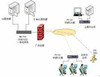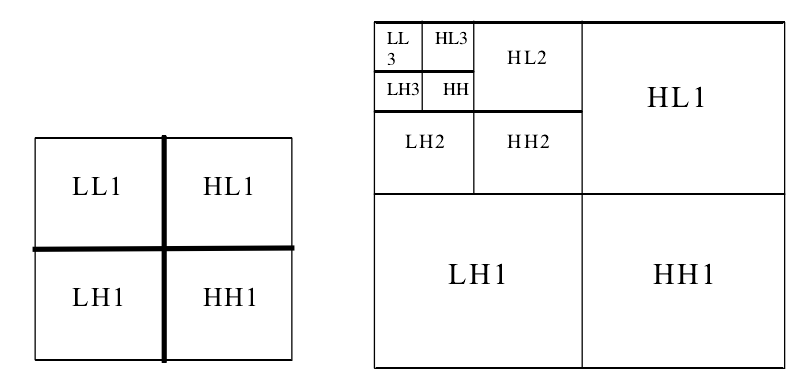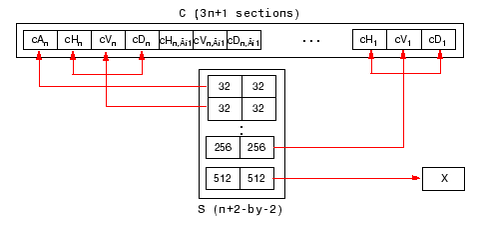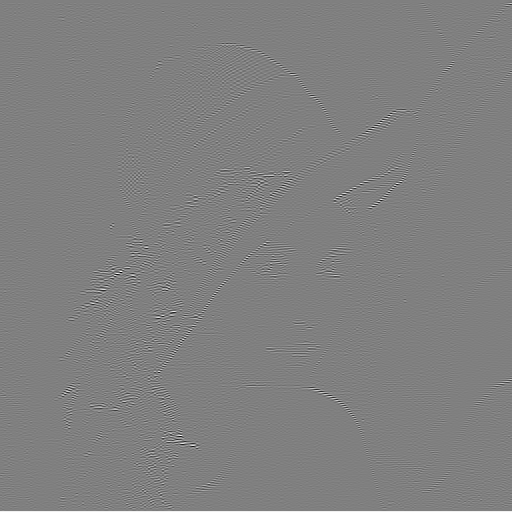tls 订阅

Transport Layer Security

TLS

TLS简介

• ## matlab图像处理

千次阅读 2017-09-22 13:07:47
matlab图像处理
matlab中 fprintf 和disp的用法

disp(‘pi=’,pi)
??? Error using ==> disp
Too many input
arguments.

disp(sprintf(‘圆周率pi= %8.5f’,pi))
圆周率pi=  3.14159

想把字符串pi=和变量pi的值显示在一行上,试用disp(′pi=′,pi),回答这是非法的.这时应该用sprintf函数,它可把数据按要求的格式转换为字符串,再把它与需要显示的字符串组装成一个长字符串,使显示格式非常灵活,人机界面更为友好.如键入
st=sprintf(′ 圆周率pi= %8.5f′,pi);
disp(st)
结果为
圆周率pi=3.14159
其中,%为数据格式符,f表示十进制浮点,8.5表示数字的长度为8位,小数点后5位.从%到f之间的字符都是不显示的,它只指出显示数据pi的格式，跟C的printf很相似。

字 符 串 转 换

abs

字符串到ASCII转换

dec2hex

十进制数到十六进制字符串转换

fprintf

把格式化的文本写到文件中或显示屏上

hex2dec

十六进制字符串转换成十进制数

hex2num

十六进制字符串转换成IEEE浮点数

int2str

整数转换成字符串

lower

字符串转换成小写

num2str

数字转换成字符串

setstr

ASCII转换成字符串

sprintf

用格式控制，数字转换成字符串

sscanf

用格式控制，字符串转换成数字

str2mat

字符串转换成一个文本矩阵

str2num

字符串转换成数字

upper

字符串转换成大写

在许多情况下，希望把一个数值嵌入到字符串中。几个字符串转换可完成这个任务。

» t=[’ A circle of radius ’ num2str(rad) ’ has an area of ’ num2str(area) ’ . ’ ] ;

» disp(t)

A circle of radius 2.5 has an area of 19.63.

这里函数num2str用来把数值转换成字符串，字符串连接用来把所转换的数嵌人到一个字符串句子中。按类似方式，int2str把整数转换成字符串。无论是num2str还是int2str都调用函数sprintf，它用类似C语言语法把数值转换成字符串。

函数fprintf经常是函数disp的一个有用替换，由于它提供了对结果更多的控制。当准备把格式化的数据写到一个文件中去时，按缺省它在命令窗口显示结果。例如，

» fprintf(’ See what this does ‘)

See what this does»

» fprintf(’ See what this does\n ‘)

See what this does

在上面第一个例子里，fprintf显示字符串，然后立即给出MATLAB提示符。相反，在第二个例子里，\n插入一个新行字符，在MATLAB提示符出现之前创建一个新行。

无论fprintf还是sprintf以同样方式处理输入参量，但fprintf把输出送到显示屏或文件中，而sprintf把输出返回到一个字符串中。例如，上面的例子用num2str可重写为

» t=sprintf(’ A circle of radius %.4g has an area of %.4g. ‘, rad, area);

» disp(t)

A circle of radius 2.5 has an area of 19.63.

» fprintf(’ A circle of radius %.4g has an area of %.4g.\n ’ , rad, area)

A circle of radius 2.5 has an area of 19.63.
这里%.4g是用在函数num2str中的数据格式。%.4g就是用指数或定点标记，不管哪一种更短些，只显示至4位数字。除了g格式，还可用e (指数)和f(定点)转换。表6.2表明在各种不同转换下，如何显示pi结果。

表6.2

数 值 格 式 转 换 例 子

命令

结果

fprintf(’ %.0e\n ‘,pi)

3e+00

fprintf(’ %.1e\n ‘,pi)

3.1e+00

fprintf(’ %.3e\n ‘,pi)

3.142e+00

fprintf(’ %.5e\n ‘,pi)

3.14159e+00

fprintf(’ %.10e\n ‘,pi)

3.1415926536e+00

fprintf(’ %.0f\n ‘,pi)

3

fprintf(’ %.1f\n ‘,pi)

3.1

fprintf(’ %.3f\n ‘,pi)

3.142

fprintf(’ %.5f\n ‘,pi)

3.14159

fprintf(’ %.10f\n ‘,pi)

3.1415926536

fprintf(’ %.0g\n ‘,pi)

3

fprintf(’ %.1g\n ‘,pi)

3

fprintf(’ %.3g\n ‘,pi)

3.14

fprintf(’ %.5g\n ‘,pi)

3.1416

fprintf(’ %.10g\n ‘,pi)

3.141592654

fprintf(’ %.8.0g\n ‘,pi)

3

fprintf(’ %.8.1g\n ‘,pi)

3

fprintf(’ %.8.3g\n ‘,pi)

3.14

fprintf(’ %.8.5g\n ‘,pi)

3.1416

fprintf(’ %.8.10g\n ‘,pi)

3.141592654

注意，对e 和f 格式，小数点右边的十进制数就是小数点右边要显示的多少位数字。相反，在g的格式里，小数点右边的十进制数指定了显示数字的总位数。另外，注意最后的五行，其结果指定为8个字符长度，且是右对齐。在最后一行，8被忽略，因为指定超过了8位。

概括起来，当需要比缺省函数disp，num2str和int2str所提供的更多的控制时，fprintf和sprintf是有用的。

函数str2mat把一列的几个字符串转换成一个字符串矩阵。例如，

» a=’ one ’ ; b= ’ two ’ ; c= ’ three ’ ;

» disp(str2mat(a, b, c, ’ four ‘))

one

two

three

four

从上面看不明显，上面的每行有同样数目的元素。较短行用空格补齐，使结果形成一个有效的矩阵

在逆方向转换中，有时是很方便的。

» s= ’ [1 2; pi 4] ’ % a string of a MATLAB matrix

s =

[1 2; pi 4]

» str2num(s)

ans =

1.0000 2.0000

3.1416 4.0000

» s=’ 123e+5 ’ % a string containing a simple number

s =

123e+5

» str2num(s)

ans =

12300000

函数str2num不能接受用户定义的变量，也不能执行转换过程的算术运算。

pcolor（Ｘ，Ｙ，Ｃ）函数的用法： X，Y是用来定位的，C是用来填充颜色的。

当X,Y是向量时，X与C的列对应，Y与C的行对应，因此向量X和Y的维数必须要求与C的列与行统一。所以使用时注意检查X,Y,C的大小和维数。

其后面最好用上函数：shading interp;
展开全文• Matlab图像处理新手——图像分割和画框显示篇 **前言：**许多人说Matlab对未来的工作没什么用，就目前了解的情况而言并非如此。如：现版本已有matlab转化成C语音的功能；matlab与DSP（API协议技术）、FPGA、西门子...
Matlab图像处理新手——图像分割和画框显示篇
**前言：**许多人说Matlab对未来的工作没什么用，就目前了解的情况而言并非如此。如：现版本已有matlab转化成C语音的功能；matlab与DSP（API协议技术）、FPGA、西门子PLC(OPC技术)等。matlab得益于他丰富的库函数。当然，他不能作为硬件的主语言，当作一种手段吧。另外，作为图像处理的新手，希望自己可以坚持写技术博客，像那些大牛一样。总结这方面的知识，方法，具体的实施。。
话不多说，第一篇记录图像分割。先说要处理的目标和要达到的效果。静态物体的分割，达到画框显示和处理后的图片。
分割方法：形态学的腐蚀膨胀。原本想用阈值法的，但是效果不理想。
程序：
I=rgb2gray(I);%灰度化
I=im2double(I);%将输入转换为double类。若输入是uint8类 uint16 类 logical类则函数将其转换为范围[0,1]之间的类，若输入是double类,则函数im2double将返回一个与输入相等的数组.
figure,imshow(I),title(‘原图’);
se=strel(‘disk’,10);%strel用于膨胀腐蚀及开闭运算等操作的结构元素对象；创建半径为10的圆
ft=imtophat(I,se);%对输入的灰度图或二值图I进行形态高帽滤波，高帽滤波计算图像的形态开启(使用imopen)，然后从原始图像中减去结果。imtophat使用结构元素SE，其中SE是由strel返回。SE必须是单一的结构元素对象，而不是一个包含多结构元素对象的数组。
Th=graythresh(ft);
Th
G=im2bw(ft,Th);
figure,imshow(G),title(‘局部阈值’);
画框显示的程序：
function [state,result]=draw_rect(img,startPosition,windowSize,showOrNot)
% 函数调用：[state,result]=draw_rect(img,startPosition,windowSize,showOrNot)
% 函数功能：在图像画个长方形框
% 函数输入：img为原始的大图，可为灰度图，可为彩色图
%          startPosition 框的左上角在大图中的坐标(每行代表x,y坐标)，startPosition=[10,30],分别表示x,y为10,30
%          windowSize 框的大小 windowSize=[112,92] 分别表示宽、高
%          showOrNot 是否要显示结果?默认为显示出来?
% 函数输出：state – 表示程序结果状态?
%          result - 结果图像数据
if nargin < 4
showOrNot = 1;
end
rgb = [255 0 0];    % 边框颜色
result = img;
[imgH, imgW] = size(img);
lineSize = 3;                                      % 边框大小，取1,2,3
windowSize(1,1)=windowSize(1,1);
windowSize(1,2) = windowSize(1,2);
if windowSize(1,2) > size(img,1) ||…
windowSize(1,1) > size(img,2)
state = -1;                                     % 说明窗口太大，图像太小，
disp(‘the window size is larger then image…’);
return;
end
if size(img,3) == 3
for k=1:3
for i=1:size(startPosition,1) %矩形框的总数
if(startPosition(i,1)>=0 && startPosition(i,2)>=0)
result(startPosition(i,2),startPosition(i,1):startPosition(i,1)+windowSize(i,1),k) = rgb(1,k); %画上边框
result(startPosition(i,2):startPosition(i,2)+windowSize(i,2),startPosition(i,1)+windowSize(i,1),k) = rgb(1,k);%画右边框
result(startPosition(i,2)+windowSize(i,2),startPosition(i,1):startPosition(i,1)+windowSize(i,1),k) = rgb(1,k);  %画下边框
result(startPosition(i,2):startPosition(i,2)+windowSize(i,2),startPosition(i,1),k) = rgb(1,k);   %画左边框
			if lineSize == 2 || lineSize == 3
result(startPosition(i,2)+1,startPosition(i,1):startPosition(i,1)+windowSize(i,1),k) = rgb(1,k);
result(startPosition(i,2):startPosition(i,2)+windowSize(i,2),startPosition(i,1)+windowSize(i,1)-1,k) = rgb(1,k);
result(startPosition(i,2)+windowSize(i,2)-1,startPosition(i,1):startPosition(i,1)+windowSize(i,1),k) = rgb(1,k);
result(startPosition(i,2):startPosition(i,2)+windowSize(i,2),startPosition(i,1)-1,k) = rgb(1,k);

if lineSize == 3
result(startPosition(i,2)-1,startPosition(i,1):startPosition(i,1)+windowSize(i,1),k) = rgb(1,k);
result(startPosition(i,2):startPosition(i,2)+windowSize(i,2),startPosition(i,1)+windowSize(i,1)+1,k) = rgb(1,k);
result(startPosition(i,2)+windowSize(i,2)+1,startPosition(i,1):startPosition(i,1)+windowSize(i,1),k) = rgb(1,k);
result(startPosition(i,2):startPosition(i,2)+windowSize(i,2),startPosition(i,1)+1,k) = rgb(1,k);
end
end
end
end
end

end
state = 1;
if showOrNot == 1
figure;
hold on;
imshow(result);
end
在这里吐槽一下，关于function的调用也是摸索了一下。。是综合了四个程序，做成自己需要的。在此有所感想，关于码程序这件事，是在诸多程序之中，找到自己想要的，综合，不断地改改改。主函数就不贴出来了，根据自己的情况写就行了。然后综合上面的算法处理部分就完成了。。
接下来要做的1、是基于动态目标的跟踪检测，算法还在研究之中，显示动态的话自然得用自动分割了。。上面的是交互式分割。2、总结出静态目标的分割方法，并码一遍代码。主要是阈值法，区域分割法和分水岭分割法。。


展开全文• 前言   从傅里叶变换到短时傅里叶变换再到小波变换，这些分析问题的方法是一代一代人的探索和积累得来的宝贵知识财富。比较常见的还有脊波变换，曲波变换，...这里主要记录MATLAB小波包中的函数的用法而已，也...
前言
从傅里叶变换到短时傅里叶变换再到小波变换，这些分析问题的方法是一代一代人的探索和积累得来的宝贵知识财富。比较常见的还有脊波变换，曲波变换，轮廓波变换。感觉一种方法弄懂了，在以后很有可能会再次用到。就像这次，本来本科毕设已经用到了小波变换和轮廓波变换，但是自己并没有把它完全弄懂，结果这次课程作业还是要重新看。。。虽然这一次也还是没搞懂。。这里主要记录MATLAB小波包中的函数的用法而已，也只记录了二维离散小波在图像分解和重构上的应用，小波变换好多基本概念自己还是没理解。
正题
图图1
二维离散小波变换最常见的应该是上面的图了。首先要明白，进行小波分解后得到的是一个低频分量和3个高频分量。其中LL1代表低频分量，HL1、HH1和LH1代表高频分量，后面的1代表是第一级分解。也经常用A（低频分量）、H（水平方向高频分量）、V（垂直方向高频分量）、D（对角线方向高频分量）来表示。
wavedec2(X,N,‘wname’)
例：[c,s]=wavedec2(X,2,'haar');
这个函数就是将一幅图像X分解成小波系数存入c中，其中N代表分解的级数，wname代表所用的小波基函数。s 记录了每一级分解时各个小波系数的size，如下图，size记录的其实就是最开始那个图上，HH1，HH2，HH3…的边长，也就是像素大小。s最后一行是原始图像的大小。detcoef2(O,C,S,N)
例：[H1,V1,D1] = detcoef2('all',c,s,1);
将c里面的高频系数重组成矩阵形式，参数N代表对第N级的分解系数进行操作。此函数只能重组高频系数,H1为水平方向，V1为垂直方向，D1为对角线方向。
appcoef2(C,S,‘wname’,N)
例：A2 = appcoef2(c,s,'haar',2);
将c里面的低频系数重组成矩阵形式,N代表第几级分解。
wrcoef2(‘type’,C,S,‘wname’,N)
例：X_A2 = wrcoef2('a',c,s,'sym5',1)
通过第N级分解的低频系数或高频系数重构图像。其中type是代表选择的是系数A，V，H，D中的哪一个进行重构。
X = waverec2(C,S,‘wname’)
例：waverec2(c_Recon1,s,'haar')
直接通过c里面的所有系数来重构图像，但这里一般会将c里面部分系数置0，然后再重构图像。如果不置0的话，不就相当于重构回来的就是原来一模一样的图像了吗。
下面是一个简单的图像重构的程序，先看一下部分图像下面是代码
%% 小波分解与重构
clear;clc;close all;
% figure,imshow(X);
[c,s]=wavedec2(X,2,'haar');%对图像X进行4级小波分解，分解得到的系数存到c，s是记录各个级系数的size
%其实感觉这里可以把排序，从大到小，当然是H，D，A，V各排各的。在重构时，丢系数时从后面往前丢。

[H1,V1,D1] = detcoef2('all',c,s,1);%将c里面的系数重组成矩阵形式，参数1代表对第一级的分解系数进行操作。都是高频系数，代表边缘
% A1 = appcoef2(c,s,'haar',1);%一级分解低频系数
[H2,V2,D2] = detcoef2('all',c,s,2);%将c里面的系数重组成矩阵形式，参数1代表对第一级的分解系数进行操作。都是高频系数，代表边缘
A2 = appcoef2(c,s,'haar',2);%二级分解低频系数

X_A2 = wrcoef2('a',c,s,'sym5',1); %通过二级分解后的低频系数重构图
X_H1 = wrcoef2('h',c,s,'sym5',1);
X_V1 = wrcoef2('v',c,s,'sym5',1);
X_D1 = wrcoef2('d',c,s,'sym5',1);
X_H2 = wrcoef2('h',c,s,'sym5',2);
X_V2 = wrcoef2('v',c,s,'sym5',2);
X_D2 = wrcoef2('d',c,s,'sym5',2);

%% 保存各级分解重构图片
figure(1);imshow(X_A2,[]);saveas(1,'picture/lena_A2.bmp');
figure(2);imshow(X_H1,[]);saveas(2,'picture/lena_H1.bmp');
figure(3);imshow(X_V1,[]);saveas(3,'picture/lena_V1.bmp');
figure(4);imshow(X_D1,[]);saveas(4,'picture/lena_D1.bmp');
figure(5);imshow(X_H2,[]);saveas(5,'picture/lena_H2.bmp');
figure(6);imshow(X_V2,[]);saveas(6,'picture/lena_V2.bmp');
figure(7);imshow(X_D2,[]);saveas(7,'picture/lena_D2.bmp');
%% 图像重构
c_RemainPortion = 0.05;%保留字带的系数所占百分比
c_end = s(1,1)^2+round((length(c)-  s(1,1)^2)*c_RemainPortion);%在将系数c的后面部分设为0时，要考虑c的最前面是低频分量，是不可以去掉的；
c_Recon1 =zeros(1,length(c));
c_Recon1(1:c_end) = c(1:c_end);
X_Recon1 = uint8(waverec2(c_Recon1,s,'haar'));
figure(8);imshow(X_Recon1,[]);title('保留系数5%');saveas(8,'picture/lena_Recon1.bmp');

c_RemainPortion = 0.1;%保留字带的系数所占百分比
c_end = s(1,1)^2+round((length(c)-  s(1,1)^2)*c_RemainPortion);%在将系数c的后面部分设为0时，要考虑c的最前面是低频分量，是不可以去掉的；
c_Recon2 =zeros(1,length(c));
c_Recon2(1:c_end) = c(1:c_end);
X_Recon2 = uint8(waverec2(c_Recon2,s,'haar'));
figure(9);imshow(X_Recon2,[]);title('保留系数10%');saveas(9,'picture/lena_Recon2.bmp');

c_RemainPortion = 0.2;%保留字带的系数所占百分比
c_end = s(1,1)^2+round((length(c)-  s(1,1)^2)*c_RemainPortion);%在将系数c的后面部分设为0时，要考虑c的最前面是低频分量，是不可以去掉的；
c_Recon3 =zeros(1,length(c));
c_Recon3(1:c_end) = c(1:c_end);
figure(10);X_Recon3 = uint8(waverec2(c_Recon3,s,'haar'));
figure(9);imshow(X_Recon3,[]);title('保留系数20%');saveas(9,'picture/lena_Recon3.bmp');

%% 计算熵和信噪比
entropy(X);
entropy(X_Recon1)
MSE1 = mean(mean(X-X_Recon1).^2);
PSNR1 = 20*log10(double(255/MSE1))
entropy(X_Recon2)
MSE2 = mean(mean(X-X_Recon2).^2);
PSNR2 = 20*log10(double(255/MSE2))
entropy(X_Recon3)
MSE3 = mean(mean(X-X_Recon3).^2);
PSNR3 = 20*log10(double(255/MSE3))




展开全文图像二值化就是将图像上的像素点的灰度值设置为0或255，也就是将整个图像呈现出明显的黑白效果的过程。

    A=imread(D:\A);
thresh=graythresh(A);%确定二值化阈值
B=im2bw(A,thresh);%对图像二值化
展开全文二值化
• 时间：2014年9月12日00:09:01 整理：天空之恋 地点：桂电
• Matlab-分割与边缘检测实验 使用一阶和二阶检测算子（导数）进行图像的边缘检测。 问题1：编写程序实现一阶Sobel算子，进行图像的边缘提取； 问题2：编写程序实现一阶Prewitt算子，进行图像的边缘提取； 问题3：...边缘检测
• 本实验为使用MATLAB编写的细胞图像分割及计数系统，实现了对图像内细胞的计数，以及对每个细胞周长和面积的测量，并分别展示了分割后的每个细胞的图像。 实验步骤共分为图像预处理、图像预分割、空洞填充、黏连细胞...
• ## matlab图像处理——平滑滤波

万次阅读 多人点赞 2018-11-01 20:52:00
平滑滤波——matlab图像处理 平滑滤波的目的是消除或尽量减少噪声，改善图像的质量。假设加性噪声是随机独立分布，这样利用图像像素领域的平均或加权平均即可有效地抑制噪声干扰。从信号分析的观点来看，图像平滑...平滑滤波
• ## Matlab实现人脸识别

万次阅读 多人点赞 2018-06-13 21:35:23
最近在学习matlab图像处理部分，发现人脸识别这一块非常好玩，在这里做个总结。人脸识别之一：查找图片中的人脸并用方框圈出 这种类似于智能手机拍照时，屏幕里那个框任务头部的红框。大致步骤为：获取RGB图片---&...人脸识别
• 一、实验名称 图像锐化 二、实验目的 ...利用图像基于单方向梯度算子，Robert算子，Sobel算 子，Pritt算 子, Laplacian算子，多方向模板，LoG算子编写matlab增强程序;最后，显示边缘图像和锐化增强...图像锐化
• ## 数字图像处理实战

千人学习 2019-06-07 12:08:03
本课程内容主要选取自stanford EE368/CS232 课程的...本次课程包含图像算法和机器学习，编程工具主要包括python，c++，android，matlab，opencv，Tesseract等。 由于个人时间精力有限，课程将每隔2-3周发布一次。基础算法 算法基础 算法设计与分析基础
• ## matlab实现图像的高斯滤波

万次阅读 多人点赞 2019-04-23 16:09:08
高斯滤波的含义：高斯滤波就是对整幅图像进行加权平均的过程，每一...高斯滤波的作用：高斯滤波是一种线性平滑滤波，适用于消除高斯噪声，广泛应用于图像处理的减噪过程。 高斯滤波函数 一维高斯分布 二维高斯分...高斯滤波
• 二值化阀值 将256个亮度等级的灰度图像通过适当的阀值选取，所有的灰度大于或等于阀值的像素被判定为属于特定物体，其灰度值为255表示，否则这些 像素点被排除在物体区域以外，灰度...在matlab中二值化图像函数为i...
• 图像直方图均衡化 首先，我们要理解什么是图像直方图均衡化： 把原始图像的灰度直方图从比较集中的某个灰度区间变成在全部灰度范围内的均匀分布。直方图均衡化就是对图像进行非线性拉伸，重新分配图像像素值，使一定...图像直方图均衡化
• （1） 检查可处理的图像文件格式： ...（3） 解析图像采集工具箱的硬件信息，这个图像处理时比较少用： imaqparsehwinfo （4） 图像的基本信息获取： 这个命令相对比较重要，它可以获得图像的各种属性。
• ## 图像入门：MATLAB图像识别

万次阅读 多人点赞 2018-07-08 15:25:14
初学数字图像处理，做一个练习，识别图中的图形形状，颜色，位置，面积，周长f=imread('C:\Users\QHJ\Desktop\demo.png'); f=f(:,:,3); f=histeq(f,256); %增强对比度 f=im2bw(f,0.386); rowhigh=102+276-1; %提取...
• OTSU算法是由日本学者OTSU于1979年提出的一种对图像进行二值化的高效算法。（大津算法） Otsu原理 对于图像 t (x,y)，前景(即目标)和背景的分割阈值记作 T，属于前景的像素点数占整幅图像的比例记为 ω0，平均灰度为...otsu算法 最大类间方差法
• matlab截取图像Ⅱ 欢迎来到！德莱联盟 应该是CSDN之bruderlung的博客！ 程序要求 建立循环函数，通过程序依此读取十幅原始图像，然后将原始图像转存为灰度图像 确定数码管所显示数字的四角坐标，然后将...matlab截图
• 今天发现了matlab图像处理工具箱的强大之处， 任务缘由： 需要对图像进行批处理改变尺寸 一般遇到这种任务，我们往往都会写matlab代码进行批量操作 但是matlab有自带的很强大的图像处理工具箱，  图片批处理...
• 软件：matlab 2014b 利用matlab绘制灰度直方图 figure; I=imread('G:\matlab\img\1.jpg'); subplot(1,2,1);imshow(I); title('原始图像'); imwrite(I,'test.png'); subplot(1,2,2); imhist(y); title('灰度图像...灰度直方图
• 图像处理 二维离散傅里叶变换DFT matlab代码图像处理领域离散傅里叶变换的作用二维离散傅里叶变换二维离散傅里叶变换公式将二维的离散傅里叶变换进行转化将系数转化为矩阵形式注意，从矩阵的乘积i形式可以看出，原来...二维离散傅里叶变换
• ## SIFT matlab源代码解析

万次阅读 多人点赞 2015-02-08 20:58:35
sift特征匹配算法可以处理两幅图像之间发生一些平移、旋转、仿射等匹配问题。因为早前自己要做一个图像拼接的问题，所以用到了sift。写这篇blog，是因为自己准备向CV进军，开始着手写blog来积累知识，这也是我第一篇...
• ## Python图像处理基础

千次阅读 多人点赞 2018-01-19 11:38:10
对我个人而言使用Python图像处理意在取代matlab，集中化使用Python环境保证之后在机器学习和OpenCV的使用上具有一致性，虽然从实验室师兄师姐的口中得知Python的图像处理较之matlab相对复杂（应该只是代码量的问题）...Python
• ## MATLAB手写数字识别

千次阅读 多人点赞 2019-07-31 14:07:22
本文主要是根据《matlab手写神经网络实现识别手写数字》博客中的代码进行试验。由于没有数据集，所以采用了MNIST数据集进行代码的运行。数据集不同所以需要对代码进行微小改动。 简介 数据处理：4000张作为训练样本...手写数字识别
• ## matlab人脸识别论文

千次阅读 多人点赞 2019-10-11 17:41:51
再由PCA算法对人脸图像进行主成分特征提取,进--步降低图像数据的处理量;最后使用经过训练后的BP神经网络对待测人脸进行分类识别。详细介绍了离散小波变换PCA特征提取以及BP神经网络分类设计。通过系统仿真实验与分析...
• ## 基于MATLAB的人脸识别算法的研究

万次阅读 多人点赞 2017-12-04 20:24:32
基于MATLAB的人脸识别算法的研究 ...今天我们将从MATLAB图像识别说起，后边将陆续讲解车牌识别，交通标牌识别等，并努力将它移植到FPGA里边做到高速实时处理MATLAB人脸识别的处理过程： 1....脸部识别 算法 机器视觉
• 图像的灰度变换与直方图修正 二、实验目的 1.熟悉MATLAB软件的使用。 2.掌握灰度变换、直方图修正的原理及数学运算。 3.于MATLAB环境下编程实现对图片的灰度变换和直方图修正。 三、实验内容 1.对于给定图片，在...灰度变换 直方图修正
• ## matlab 均值滤波

千次阅读 2018-11-21 15:44:02
但是想学习图像处理的滑，是远远不够的，还要对算法的基本原理弄清楚 1-均值滤波 均值滤波也叫做线性滤波，规定一个固定可调节大小的模板，如3*3，对目标像素做均值滤波就是将他周围8个点的像素值求和取平均，替代...
• ## 基于Matlab的车牌识别系统

千次阅读 热门讨论 2019-03-26 21:01:06
研究内容：基于Matlab车牌图像识别的设计与实现是使用Matlab图像处理和获取工具箱，获取静态车牌图像并进行处理，依据图像特点还原车牌文字信息 研究方法：利用matlab对车牌图像进行定位与分割等处理 在Matlab中通过...车牌识别 车牌定位 字符分割
• ## matlab实现人脸识别

万次阅读 2015-09-08 12:30:03
人脸识别技术中被广泛采用的区域特征分析算法，它融合了计算机图像处理技术与生物统计学原理于一体，利用计算机图像处理技术从视频中提取人像特征点，利用生物统计学的原理进行分析建立数学模型,即人脸特征模板。...人脸识别 最小距离法人脸识别...